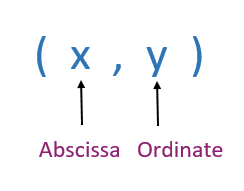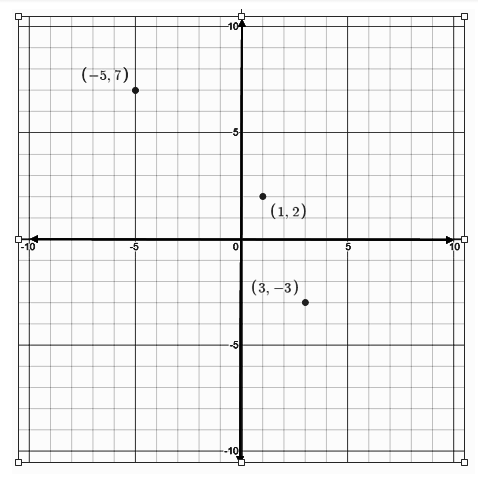# Ordinate

In the coordinate system, the term “Ordinate” refers to the y -coordinate in  (x, y). In short, the distance along the y-axis is called the ordinate. There is a difference in two terms called coordinate and ordinate. The term “coordinate” represents the position of the point along the line, arc and so on, whereas the term “ordinate” represents the value of a coordinate on the y-axis. In this article, we are going to discuss what is ordinate in Math, what is abscissa and ordinate with an example, and how to plot the ordinates in the coordinate system in detail.

## Ordinate MeaningThe ordinate is the second component of an ordered pair. For example, if (x, y) is an ordered pair, then y is the ordinate here. An ordered pair is used to denote a point in the Cartesian plane. It is the second of the two terms (x, y) by which a point is defined, in a system of fixed Cartesian Coordinate plane. The first coordinate (x), in the plane, is called the abscissa. The Cartesian system designates each point in the XY plane, uniquely, by a pair of numerical coordinates. These points are at the distances from the two fixed axes, measured in the same unit of length.

### Ordinate Example

Take an example of a point A in the Cartesian plane, having coordinates (6, 9), where 9 is the ordinate and a point B, having coordinates (7, -5), where -5 is the ordinate. In case of point A, the ordinate is positive, so we will move towards a positive direction on the y-axis from the origin (0, 0) at 9 equal divisions but in case of point B, we will move towards negative direction at 5 equal divisions.

## Ordinate and Abscissa

The distance of a point from y-axis scaled with the x-axis is called abscissa or x coordinate of the point. The distance of a point from x-axis scaled with the y-axis is called ordinate. The abscissa and ordinate all together are called coordinates.

The coordinates are written in the brackets (), where abscissa is written in the first position and then ordinate in the second position, separated by a comma. If x and y are abscissa and ordinate respectively, then the location of the point P in the Cartesian plane is expressed by the ordered pair (x, y).

## Plotting Ordinates in Coordinate System

The graph given below is an example of a Cartesian coordinate system, having 3 different points marked in the XY plane.The 3 points (1, 2), (3, -3) and (-5,7) are away from the origin (0, 0). For the point (1, 2), while plotting the ordinate, two parts are covered in the positive direction of the Y-axis. Similarly, for point (3, -3) the ordinate cover three parts towards the negative direction of Y-axis, as the ordinate is negative here.

### Relation of Ordinate in the Coordinate System

The ordinate depends entirely on the Y-axis. So while plotting it in a Cartesian plane, we have to move along or parallel to the positive or negative direction of the Y-axis. This represents the relation between the coordinate system and the y-axis.

To learn more terminology in the coordinate system and other topics, register with BYJU’S – The Learning App and download the app to learn with ease.